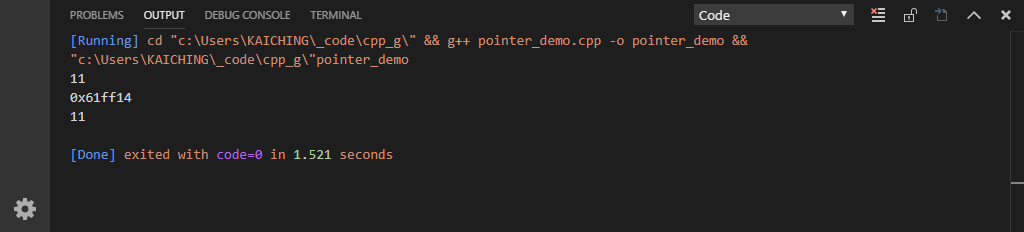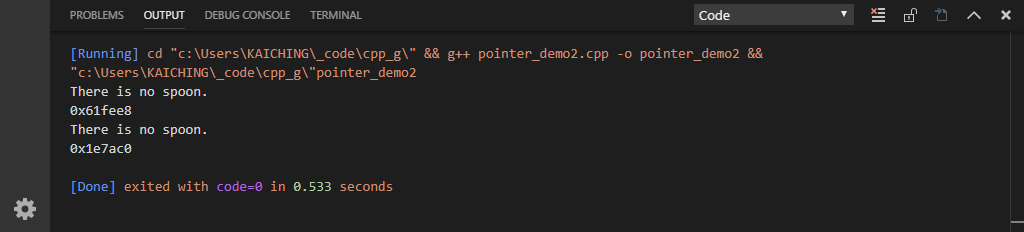# 單元 4 - 指標與參考

*nPtr &nRef

C++ 中，除了基本內建型態指標外，其他的變數大都可看作是物件，指標是承接自 C 語言儲存記憶體位址的概念，如果指標運用得宜就可以寫出相當有效率的程式。

``````#include <iostream>

using namespace std;

int main(void) {
// 設定 n 為整數 11
int n = 11;
cout << n << endl;

// 設定 nPtr 為指向 n 的指標
int *np = &n; // & 是取址運算子
cout << np << endl;

// 設定 t 取得從 np 位址指向的值
int t = *np; // * 是反參考運算子
cout << t << endl;

return 0;
}

/* 《程式語言教學誌》的範例程式
http://kaiching.org/
檔名：pointer_demo.cpp
功能：示範指標運算子
作者：張凱慶 */``````

``````// 設定 n 為整數 11
int n = 11;
cout << n << endl;``````

``````// 設定 nPtr 為指向 n 的指標
int *np = &n; // & 是取址運算子
cout << np << endl;``````

``````// 設定 t 取得從 np 位址指向的值
int t = *np; // * 是反參考運算子
cout << t << endl;````````````#include <iostream>
#include <string>

using namespace std;

int main(void) {
// a 為字串變數
string a = "There is no spoon.";
cout << a << endl;

// b 為對 a 的指標
string *b = &a;
cout << b << endl;

// c 為對 a 的參考
string &c = a;
cout << c << endl;

// d 為另一個指標
string *d = new string("There is no spoon.");
cout << d << endl;

return 0;
}

/* 《程式語言教學誌》的範例程式
http://kaiching.org/
檔名：pointer_demo2.cpp
功能：示範指標及參考運算子
作者：張凱慶 */``````

``````// c 為對 a 的參考
string &c = a;
cout << c << endl;``````

``````// d 為另一個指標
string *d = new string("There is no spoon.");
cout << d << endl;``````

new指標為建立物件的另外一種方式，此例編譯執行結果如下1. 指標用來儲存記憶體位址，宣告指標變數使用 * 運算子，可用取址運算子 & 取得記憶體位址。
2. 參考是變數的別名，宣告參考使用 & 符號。
3. 若用指標指向物件，新建物件須用 new 關鍵字及建構函數初始化物件，字串則可用字面常數的形式直接指派。

1. 為什麼指標運用得宜，可以寫出相當有效率的程式？
2. 反參考運算子用於指標所指向變數的值，是否也可以用於設定指標所指向變數的值呢？
3. 參考的用途為何？指標跟參考可以互相替代嗎？

1. 寫一個程式 exercise0401.cpp ，先宣告一個 int 型態的變數 a ，並將 a 的值設定為 1 ，然後用指標 pa 取得 a 的記憶體位址。
2. 承上題，另寫一個程式 exercise0402.cpp，除了加入 exercise0401.cpp 的內容外，繼續加入一行 *pa = 2; ，觀察 a 的值是否有改變。
3. 承上題，另寫一個程式 exercise0403.cpp，除了加入 exercise0402.cpp 的內容外，繼續加入一個參考變數 ra ，以 ra 當作 a 的別名。
4. 承上題，另寫一個程式 exercise0404.cpp，除了加入 exercise0403.cpp 的內容外，並將 ra 更改為 3 ，觀察 a 的值是否有改變。